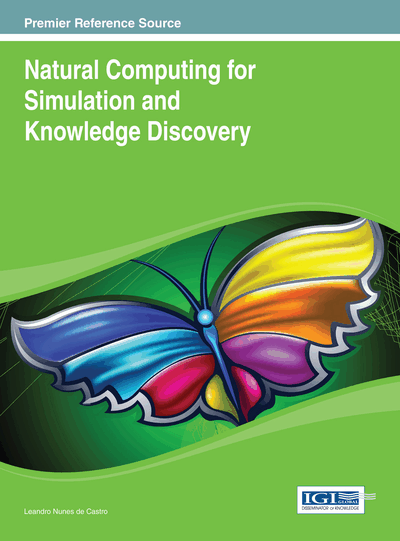# Linear Time Solution to Prime Factorization by Tissue P Systems with Cell Division

Xingyi Zhang (Anhui University, China), Yunyun Niu (Huazhong University of Science and Technology, China), Linqiang Pan (Huazhong University of Science and Technology, China) and Mario J. Pérez-Jiménez (University of Sevilla, Spain)
DOI: 10.4018/978-1-4666-4253-9.ch014

## Abstract

Prime factorization is useful and crucial for public-key cryptography, and its application in public-key cryptography is possible only because prime factorization has been presumed to be difficult. A polynomial-time algorithm for prime factorization on a quantum computer was given by P. W. Shor in 1997. In this work, it is considered as a function problem, and in the framework of tissue P systems with cell division, a linear-time solution to prime factorization problem is given on biochemical computational devices – tissue P systems with cell division, instead of computational devices based on the laws of quantum physical.
Chapter Preview
Top

## 1. Introduction

In math, prime factorization is the breaking down of a composite number into smaller primes, which when multiplied together equal the original integer. Currently, though the prime factorization problem is not known to be NP-hard, no efficient algorithm is publicly known. It is generally considered intractable. The presumed computational hardness of this problem is at the heart of several algorithms in cryptography such as RSA (Rivest, Shamir, & Adleman, 1978).

Many areas of mathematics and computer science have been brought to bear on the prime factorization problem, including elliptic curves, algebraic number theory, and quantum computing. A polynomial-time algorithm for prime factorization on a quantum computer was given by Shor in 1997. This will have significant implications for cryptography if a large quantum computer is ever built. However, before a practical quantum computer appears, it is still of interest to find any reasonable computational devices for solving the prime factorization problem. In this work, we shall give a linear-time solution to prime factorization on a class of biochemical computational devices – tissue P systems with cell division, instead of computational devices based on the laws of quantum physical.

Tissue P systems with cell division is a class of computational devices in membrane computing. Membrane computing is an emergent branch of natural computing, which is inspired by the structure and the functioning of living cells, as well as the organization of cells in tissues, organs, and other higher order structures. The devices in membrane computing, called P systems, provide distributed parallel and non-deterministic computing models. Since Păun (2000) introduced the first P system, this area is heavily investigated. Please refer to the monographs of membrane computing (Păun, Rozenberg, & Salomaa, 2010; Frisco, 2009) for general information in this area, and to the membrane computing web site (P systems web page) for the up-to-date information.

Informally, a P system consists of a membrane structure, in the compartments of which one places multisets of objects which evolve according to given rules in a synchronous, non-deterministic, maximally parallel manner. Tissue P systems are a class of P systems, where membranes are placed in the nodes of a graph. It is a net of processors dealing with objects and communicating these objects along channels specified in advance. The communication among cells is based on symport/antiport rules, which was introduced to P systems in Păun and Păun (2002). Symport rules move objects across a membrane together in one direction, whereas antiport rules move objects across a membrane in opposite directions. This model has two biological inspirations (Martín-Vide, Pazos, Păun, & Rodríguez-Patón, 2003): intercellular communication and cooperation between neurons. In Păun, Pérez-Jiménez, and Riscos-Núñez (2008), tissue P systems are endowed with the ability of producing new cells based on the mitosis or cellular division, thus obtaining the ability of generating an exponential amount of workspace in polynomial time. Such variant of tissue P systems is called tissue P systems with cell division.

## Complete Chapter List

Search this Book:
Reset